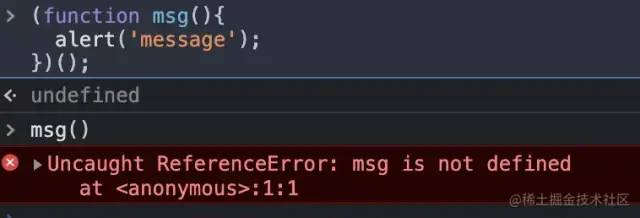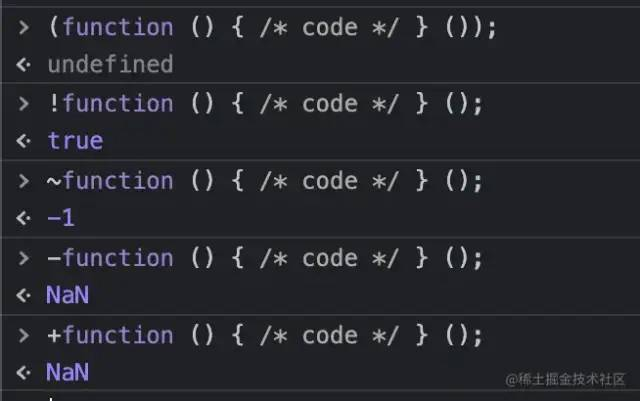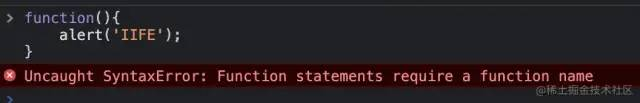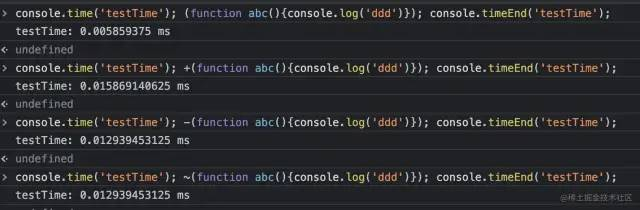# Explore why in JS, you need to add in front of the function!

Click on the top Programmer Growth Guide, pay attention to the official account

## Introduction

We basically know that there are two ways to declare a function

```function msg(){alert('msg');}//declarative definition function
Copy Code```
```var msg = function(){alert('msg');}//function assignment expression defines function
Copy Code```

But there is actually a third way of declaration, the Function constructor

```var msg = new function(msg) {
}
Copy Code```

Equivalent to

```function msg(msg) {
}
Copy Code```

The method of calling the function is usually `method name()`
However, if we try to append `()` to the end of a “definition function”, the parser cannot understand it.

```function msg(){
}();//The parser can't understand
Copy Code```

The calling method of defining a function should be `print()`; Then why it is enough to wrap the function body with `()`?
It turns out that uses parentheses to define the function body, and the parser will call the defined function in the form of a function expression. In other words, any method that can turn a function into a function expression can make the parser call the defined function correctly. And `!` is one of them, and ` + \- || ~` has such a function.

But please note that if you wrap the function body with parentheses, then execute it immediately. This method is only suitable for calling the function once, which involves a scope problem. When you want to reuse the function, the following problems will occur:But if you want to reuse the function, you can declare the function first, and then call the function. In the same parent scope, you can reuse the function, as follows:

```var msg = function(msg) {}
msg();
Copy Code```

This issue will be further analyzed later

## Self-executing anonymous function:

In many js codes, we often see such a way of writing:

```(function( window, undefined ) {
//code
})(window);
Copy Code```

We call this way of writing self-executing anonymous function. Just like its name, it executes itself. The first parenthesis is an anonymous function, and the latter parenthesis means immediate execution

As mentioned earlier, ` + \- || ~` these operators also have this function

```(function () { /* code */ } ());
!function () { /* code */ } ();
~function () { /* code */ } ();
-function () { /* code */ } ();
+ function () { /* code */ } ();
Copy Code```① ( ) has no practical meaning, does not operate the return value

② ! Negate the true and false of the return value

③ Bitwise inversion of the return value (the bitwise inversion of all positive integers is the negative number of itself + 1, the bitwise inversion of all negative integers is the absolute value of itself + 1, and the bitwise inversion of zero is -1. Among them, the bitwise inversion will also force the return value, converting the string 5 to the number 5, and then bitwise inversion. False is converted to 0, true is converted to 1. Other non-numbers Or the return value that cannot be converted into a digital type, it will be treated as 0 uniformly)

④ ~ +, – is to perform mathematical operations on the return value (it can be seen that when the return value is not a number type, +, – will perform forced conversion on the return value, and the string will be NaN after the forced conversion)

Let’s start with `IIFE` (`Note: This example is for reference on the Internet`)

IIFE (Immediately Invoked Function Expression)

```function(){
}
Copy Code```

Putting this code in `console` will result in an errorBecause this is an anonymous function, in order to make it work properly, you must give a function name, and then call it through the function name.
In fact, after adding these symbols in front of the anonymous function, turns a function declaration statement into a function expression, and the expression will be automatically executed in the `script` tag .

So now after many codes are compressed and compiled, the exported js files are usually as follows:

```(function(e,t){"use strict";function n(e){var t=e.length,n=st.type(e);return st.isWindow(e)?!1 :1===e.nodeType & amp; & amp;t?!0:"array"===n||"function"!==n & amp; & amp;(0=== t||"number"==typeof t & amp; & amp;t>0 & amp; & amp;t-1 in e)}function r(e){var t=Tt[e]={} ;return st.each(e.match(lt)||[],function(e,n){t[n]=!0}),t}function i(e,n,r,i){if( st.acceptData(e)){var o,a,s=st.expando,u="string"==typeof n,l=e.nodeType,c=l?st.cache:e,f=l ?e[s]:e[s] & amp; & amp;s;if(f & amp; & amp;c[f] & amp; & amp;(i||c[f].data)|| !u||r!==t)return f||(l?e[s]=f=K.pop()||st.guid + + :f=s),c[f]||(c [f]={},l||(c[f].toJSON=st.noop)),("object"==typeof n||"function"==typeof n) & amp; & amp;(i?c[f]=st.extend(c[f],n):c[f].data=st.extend(c[f].data,n)),o=c[f] ,i||(o.data||(o.data={}),o=o.data),r!==t & amp; & amp;(o[st.camelCase(n)]=r) ,u?(a=o[n],null==a & amp; & amp;(a=o[st.camelCase(n)])):a=o,a}}function o(e,t, n){if(st.acceptData(e)){var r,i,o,a=e.nodeType,u=a?st.cache:e,l=a?e[st.expando]:st.expando ;i f(u[l]){if(t & amp; & amp;(r=n?u[l]:u[l].data)){st.isArray(t)?t=t.concat(st .map(t,st.camelCase)):t in r?t=[t]:(t=st.camelCase(t),t=t in r?[t]:t.split(" ") );for(i=0,o=t.length;o>i;i ++ )delete r[t[i]];if(!(n?s:st.isEmptyObject)(r))return}( n||(delete u[l].data,s(u[l]))) & amp; & amp;(a?st.cleanData([e],!0):st.support.deleteExpando||u !=u.window?delete u[l]:u[l]=null)}}}function a(e,n,r){if(r===t & amp; & amp;1===e .nodeType){var i="data-" + n.replace(Nt,"-\$1").toLowerCase();if(r=e.getAttribute(i),"string"== typeof r){try{r="true"===r?!0:"false"===r?!1:"null"===r?null: + r + \ ""===r? + r:wt.test(r)?st.parseJSON(r):r}catch(o){}st.data(e,n,r)}else r=t}return r}function s(e){var t;for(t in e)if(("data"!==t||!st.isEmptyObject(e[t])) & amp; & amp;" toJSON"!==t)return!1;return!0}function u(){return!0}function l(){return!1}function c(e,t){do
Copy Code```

Operator

Some people may wonder why operators can translate declarative functions into function expressions. This involves a concept parser

The program needs to be compiled or interpreted before running, and the source program is translated into bytecode, but before the translation, the program source code in the form of a string needs to be parsed into a data structure such as a syntax tree or an abstract syntax tree, which requires the use of to the parser

## So what is a parser?

The so-called parser (Parser) generally refers to the process of converting text (string) in a certain format into a certain data structure. The most common parser (Parser) is to convert the program text into a data structure called an abstract syntax tree (AST) inside the compiler, which is also called a parser (Parser). There are also some simple parsers (Parser) for processing CSV, JSON, XML and other formats

When the JS parser performs the first step of pre-parsing, it will search from the beginning to the end of the code, and only look for content such as var, function, and parameters. The first step is generally called “JavaScript pre-parsing”. Moreover, when these contents are found, all variables are assigned a value in advance before the code is officially run: undefined; all functions are the entire function block before the code is officially run. For the parser to recognize that it is an expression, special symbols must be added to allow the parser to recognize it, such as the special operators just mentioned.

The parsing process is roughly as follows:

1. “Find something”: var, function, parameter; (also known as pre-parsing)

Remarks: If you encounter duplicate names, there are two situations: if you encounter variables and functions with duplicate names, only the function will be left; if you encounter functions with duplicate names, according to the context order of the code, keep the last one.

2. Interpret the code line by line.

Remarks: Expressions can modify the pre-parsed value (you can check the documentation yourself, this is what will be mentioned later)

## Function declaration and function definition

Function declaration The generally relatively standardized declaration form is: fucntion msg(void) Note that there is a semicolon

```function msg()
Copy Code```

Function definition function msg()Note there is no semicolon

```{
}
Copy Code```

## Function call

This is a function call

```msg();
Copy Code```

Add a `()` to the function declaration to call the function

```function msg(){
}()
Copy Code```

that’s all
But we executed it in `console` according to the above and found that something went wrongBecause such code confuses function declaration and function call, the function `msg` declared in this way should be called as `msg()`.

If it is changed to `(function msg())()`, it will be such a structure: (function body) (IIFE), which can be recognized by the Javascript parser and executed normally

Learned from the pre-parsing process of the Js parser:

Parsers recognize a pattern: use parentheses to wrap functions. This is almost always a positive signal to the parser that the function needs to be executed immediately. If the parser sees an opening parenthesis followed by a function declaration, it will immediately parse the function. You can help the parser speed up parsing by explicitly declaring functions that execute immediately

That is to say, the function of parentheses is to declare a function, let the parser recognize it as an expression, and finally execute the function by the program

## Summary

Any method that disambiguates between a function declaration and a function expression can be correctly recognized by the Javascript parser.

Assignment, logic, even commas, various operators, as long as the parser supports and recognizes special symbols can be used as a way to disambiguate, and `!function()` and `(function())`, is a way to convert it into an expression.

## Test

As for which one to use first, `()` is recommended, and other operators, compared with one more execution step, such as + (expression), that is, immediately execute the + operator operation, roughly estimated one time:## Conclusion

From the screenshot of the test results, we can roughly see that the (IIFE) method is one level faster than the operator (speed of further digits), if the time complexity of immediate execution () is O(n) , then the operator is O(10n). Of course, this is only a rough test, and the parsing speed of existing browsers, the time base is so small that it can be ignored, so depending on personal needs, the way of writing is radish and cabbage. Everyone has their own well, it depends on the individual

```Node Community